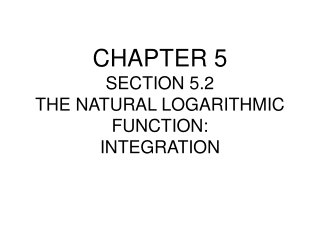DownloadDownload PresentationCHAPTER 5 SECTION 5.2 THE NATURAL LOGARITHMIC FUNCTION: INTEGRATION

# CHAPTER 5 SECTION 5.2 THE NATURAL LOGARITHMIC FUNCTION: INTEGRATION

Télécharger la présentation## CHAPTER 5 SECTION 5.2 THE NATURAL LOGARITHMIC FUNCTION: INTEGRATION

- - - - - - - - - - - - - - - - - - - - - - - - - - - E N D - - - - - - - - - - - - - - - - - - - - - - - - - - -
##### Presentation Transcript

1. CHAPTER 5SECTION 5.2THE NATURAL LOGARITHMIC FUNCTION:INTEGRATION

2. Theorem 5.5 Log Rule for Integration

3. Theorem:

4. Theorem:

5. Using Long Division Before Integrating Use of the log rule is often in disguised form Do the division on this integrand and alter it's appearance

6. Using Long Division Before Integrating • Now take the integral

7. Change of Variables • Consider • Then u = x – 1 and du = dx • But x = u + 1 and x – 2 = u – 1 • So we have • Finish the integration

8. Guidelines for Integration

9. Integrals of the Six Basic Trigonometric Functions

10. Explanations:

11. Explanations: